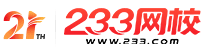#计算机二级

•扫描二维码进入

• 题 库扫描二维码进入

• 移动233网校移动

• 移动APP扫描二维码下载APP

## 2019年3月计算机二级MySQL强化练习及答案(2)

1.下列函数的类型是( )。

fun(double x)

{ float y;

y=3*x-4：

return y;

}

A.int

B.不确定

C.void

D.float

2.下列程序的运行结果是( )。

int y=5，x=14;

y=((x=3*Y，x+1)，x-1);

printf("x=%d，y=%d"，x，y);

A.x=27，y=27

B.x=12，y=13

C.x=15，y=14

D.x=y=27

3.若有下列定义和语句：

int u=011，v=0x11，w=11;

printf("%o，%x，%d\n"，u，v，w);

A.9，17，11

B.9，11，11

C.11，11，11

D.11，17，11

4.若有下列定义(设int类型变量占2个字节)：

int i=8，j=9;

printf("i=%%d，j=%%d\n"，i，j);

A.i=8，j=9

B.i=%d，j=%d

C.i=%8，j=%9

D.8，9

5.下列程序的输出的结果是( )。

main()

{ double d=3.2;int x，Y;

x=1.2;v=(x+3.8)/5.0;

printf("%d\n"，d*y);

}

A.3

B.3.2

C.0

D.3.07

6.设“int x=2，y=1;”，则表达式(!x|| Y--)的值是( )。

A.0

B.1

C.2

D.-1

7.设有以下语句：

int a=1，b=2，c;

c=a^(b<<2);

A.6

B.7

C.8

D.9

8.下列程序执行后的输出结果是( )。

void func(int*a，int b[])

{ b=*a+b;}

main()

{ int a，b;

a=0.b=3;

func(&a，b);printf("%d\n"，b);

}

A.6

B.7

C.8

D.9

9.有以下程序：

main()

{ int i，j，x=0;

for(i=0;i<2;i++)

{ x++;

for(j=0;j<=3;j++)

{if(j=0;j<=3;j++)

x++;

}

x++;

}

printf("x=%d\n"，x);

}

A.x=4

B.x=8

C.x=6

D.x=12

10.若有以下程序段，w和k都是整型变量：

w=k：

LB：if(w==0)go to LE;

W--：

printf("*")

goto LB;

LE;

A.for(w=k;w!=0;w--)printf("*");

B.for(w=k;w;--w)printf("*");

C.w=k;

while(w--!=0)printf("*");

D.w=k：

w++;do{w--;printf("*");}while(w!=0);

• 海量免费模拟试题，在线测试。

• 各科每年真题试卷，实战演练！

• 逐章逐节针对练习，专项突破。

• 每天10道最新试题，随机练习。

• QQ群号：806931213

扫描二维码加群• ##### 微信订阅号：kszx233

扫描二维码加群